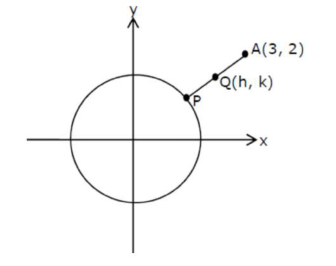# If the locus of the mid-point of the line segmentQuestion:

If the locus of the mid-point of the line segment from the point $(3,2)$ to a point on the circle, $x^{2}+y^{2}=1$ is a circle of the radius $r$, then $r$ is equal to:

1. (1) $\frac{1}{4}$

2. (2) $\frac{1}{2}$

3. (3) 1

4. (4) $\frac{1}{3}$

Correct Option: , 2

Solution:$\therefore P \equiv(2 h-3,2 k-2) \rightarrow$ on circle

$\therefore\left(h-\frac{3}{2}\right)^{2}+(k-1)^{2}=\frac{1}{4}$

$\Rightarrow$ radius $=\frac{1}{2}$